PolymathPlus is a generic mathematical problem solver. The problems are entered in a simple text format; and pressing the ► Solve button yields a detailed numerical solution report.

 Linear Equations Nonlinear Equations Ordinary Differential Equations Curve Fit Regression Variables Functions Settings and Hints Global Settings Linear Equations Hints Nonlinear Equations Hints Ordinary Differential Equations Hints Curve Fit Regression Hints

Students, engineers, scientists, or anyone with a need for a numerical math problems-solving package will appreciate the simplicity, efficiency, and speed of PolymathPlus.

The following problem types are supported:

1. Linear Equations (LEQ)
2. Nonlinear Equations (NLE)
3. Ordinary Differential Equations (DEQ)
4. Curve Fitting Regression (REG)

# Problem Entry

Problems are entered in plain text.
The # sign indicates a comment; hence any text section following it is ignored by the solution algorithm. When saving problems online, the program name is derived from the first comment line.

## System of Linear Equations  👆

The number of equations must be equal to the number of unknowns. All the algebraic expressions must be linear hence only the plus, minus, and multiplication operators are allowed. Expressions like `x*x`, `1/x`, `sin(x)` are not allowed.

The following example demonstrates the input of a linear system of equations:

Given the following set of 3 linear equations to be solved: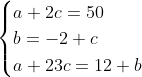this would be the data entry in the program:

# System of three linear equations a + 2*c = 50 b = -2 + c a + 23*c = 12 + b

The Solution Report includes the values of the unknowns at the solution (a = 54, b = -4, and c = -2, for this problem) and the problem definition in both matrix and system of equations formats.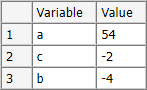## System of Nonlinear Equations  👆

When solving simultaneous nonlinear equations, the equations have to be arranged as root expressions (expressions that are equal to zero at the solution). In addition, an initial guess should be provided for each nonlinear variable.

Below is an example for solving 2 nonlinear equations with an initial estimate for x, and y at the solution.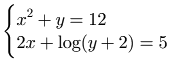Rearranging the equations, and estimating `x` and `y` to be 1 at the solution, this would be the data entry to solve this problem:

f(x) = x^2 + y - 12 f(y) = 2*x + log(y+2) - 5 x(0)=1 y(0)=1

you may define any number of auxiliary expressions, to simplify long expressions:

f(x) = x^2 + y - 12 f(y) = 2*x + a a = log(y+2) - 5 x(0)=1 y(0)=1

and the resulting solution report will show: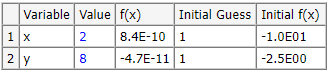PolymathPlus can numerically solve either a single nonlinear equation or a set of simultaneous nonlinear equations. When solving a single nonlinear equation, the user has to provide a range of values within which the solution exists. The program will find all the solutions within that range. When solving multiple nonlinear equations, the user has to provide a guess for each variable to be found. In this case the guess is a single value and not a range as indicated on the single nonlinear equation.
The nonlinear equations have to be arranged such that all terms are on one side and the other side should be set to zero, essentially building the root expression. The algorithm will search the independent variables which cause the root expression to become zero, or very close to zero. Additional auxiliary variables can be defined to simplify expressions for repetitive terms.

The problems belonging to this category contain implicit nonlinear algebraic equations that require an iterative solution and explicit algebraic equations where individual variables can be expressed as explicit functions of other variables and constants. An implicit nonlinear equation must be entered in the form:

f(x) = an expression

where `x` is the chosen variable name, and ` f(x)`  is an expression that will have the value of zero at the solution.

An explicit equation should be rewritten in the form:

`x = an expression `

where the expression may contain constants, implicit variables, and explicit variables.
Only real roots (non-complex) can be found. Multiple roots are determined for a single equation where the range of the variable must be provided.

## Explicit Algebraic Equations  👆

This type of problem contains a set of explicit algebraic equations. Each equation must start with a variable name followed by the equal sign and a linear or nonlinear algebraic expression. The equations may be entered in any order.  During the solution, the equations are automatically ordered to achieve a solution.  The following system of explicit equations calculates the two roots of a quadratic equation.  Note that both problem setups have the same solution.

# Explicit System of Nonlinear Equations a = 1 b = -12 c = 35 disc = b ^2-4*a* c x1 = (-b+sqrt(disc))/(2*a) x2 = (-b-sqrt(disc))/(2*a)

The Solution Report includes the values of the unknowns (x1 = 7, x2 = 5) and all the variables at the solution.

Note that the equations do not need to be ordered for solution.  Keep this option in mind for your general explicit calculational needs.

## Single Nonlinear Equation  👆

This option involves the solution of a single implicit nonlinear equation with any number of explicitly defined variables. The implicit nonlinear equation must be arranged to an expression which is equal to zero at the solution.
For the variable associated with the implicit equation, both minimal and maximal initial estimates must be provided. Multiple roots are reported if the problem has several solutions (roots) between the minimal and maximal initial estimates.
The equations and initial estimates can be entered in any order. PolymathPlus always reorders the equations during the problem solution.

Solve a nonlinear equation to find the value of x: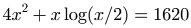The solution is expected to be found between the values 0 - 100.
The problem to enter into PolymathPlus would be:

# Solve nonlinear equation f (x) = 4*x^2 + x*log(x/2) - 1620 x (min) = 0 x (max) = 100

note that we can also define an explicit equation for the variable t that can be used to shorten large equations or ones with repeated section.

# Solve nonlinear equation f (x) = 4*x^2 + t - 1620 t = x*log(x/2) x (min) = 0 x (max) = 100

The Solution Report includes the value/s of the unknown (x=20, in this case), the function value/s (f(x)= 1.0e-8), and the values of all the variables at the solution.

## Nonlinear Equations  👆

This option involves the solution of a system comprising of several, implicit, nonlinear algebraic equations with any number of explicitly defined variables. All the implicit equations must be rewritten in the form:

f(x) = an expression

where x is a variable that does not appear on the left hand side of any of the explicit equations and the "expression" must be equal to 0 at the solution. Initial estimates must be provided for all the implicit variables using the syntax: x(0) = a value. The equations may be written in any order.

Example, Solving a set of 2 nonlinear equations: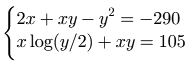The equations can be entered in the following manner (note that lines starting with # indicate user comments and are being ignored by the program):

# Solve a system of two nonlinear equations f(x) = 2*x+x*y-y^2 + 290 f(y) = t+x*y - 105 t = x*log(y/2) x(0) = 2 y(0) = 10

The Solution Report includes the values of the unknowns (x = 5, y = 20), the function values (f(x)= -2.7E-12, f(y)= 1.3E-12 ) and the values of all the additional variable/s at the solution.

## Simultaneous Linear and Nonlinear Equations  👆

A set of simultaneous linear and nonlinear equations can be solved by considering the entire set as nonlinear equations. However, if it is possible to isolate some of the variables and enter them as an explicit expression of other nonlinear variables, we would reduce the number of nonlinear variables to which we need to provide an initial guess, and as a result we would also need to arrange less expressions in a root form (expression which equals to zero). As we get fewer nonlinear equations, we increase the odds of getting a successful solution.

Consider a problem containing three linear and two nonlinear equations in the original form: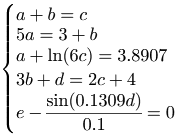These can be solved as a set of 5 nonlinear equations where an initial guess should be provided for each variable.

# Simultaneous nonlinear equations f(c) = 3.8907-ln(6*c)-a f(a) = a + b - c f(b) = 5*a - 3 - b f(d) = 2*c + 4 - 3*b - d f(e) = sin(0.1309*d)/0.1 - e # Initial guess of the variables per nonlinear equation a(0) = 2 b(0) = 2 c(0) = 2 d(0) = 2 e(0) = 2

The program text above would successfully solve the equations.
We could also rewrite the set of equations as two nonlinear equations (root expressions that are set to zero at the solutions), and three explicit expressions. This approach yields:

# Simultaneous nonlinear equations and explicit variables f(c) = 3.8907-ln(6*c)-a f(a) = a + b - c # The explicit variables b = 5*a - 3 d = 2*c + 4 - 3*b e = sin(0.1309*d)/0.1 # Initial guess of the nonlinear equation variables c(0) = 2 a(0) = 2

Note that both programs give the same solution, but the second program requires initial estimates for variables a and c only. The solution is calculated as: a = 1.00011; b = 2.00055; c= 3.00066; d = 3.99967; and e = 4.99964.

## Constrained Nonlinear Equations  👆

A special solution algorithm is available for problems where some of the variables can have only positive values.
Consider the following set of equations: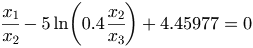having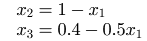In this problem, the variables x1, x2 and x3 are constrained. At the solution x1>0 because of physical considerations; x2 and x3 must be greater than zero throughout the solution process otherwise a negative value inside the ln function will yield an execution error.
Constraints allowed on the variables of the type x>0 or x<0.
For the solution, the equations for x2 and x3 must be rewritten in the implicit form as shown below:

f(x1) = x1/x2 - 5*ln(0.4*x2/x3) + 4.45977 f(x2) = x2 + x1 - 1 f(x3) = x3 + 0.5*x1 - 0.4

A solution can be achieved by using a constrained solution algorithm which is done by adding the following hint to the program (at any line location):

`#@ NLE_SOLUTION_METHOD_INDEX = 3`

The options for constrains of x1 are 'Any value' that indicates no constraints, 'positive' that means it must be positive at the solution only and 'always positive' that forces the variable to be positive all the way to the solution. For x1 the 'positive' definition is sufficient. Since the variables x2 and x3 must be positive for all calculation leading to the solution, the option 'Always positive' must be marked. The initial guesses for x2 and x3 are 0.5 and 0.15 respectively, which are consistent with initial guess of x1=0.5.
PolymathPlus designates the constrained variables in the initial guess line as follows: Positive value will have ">= 0" added to the initial guess value, Always positive values will have "> 0" added to the initial guess value.

The entire problem should look like this:

f(x1) = x1/x2 - 5*ln(0.4*x2/x3) + 4.45977 f(x2) = x2 + x1 - 1 f(x3) = x3 + 0.5*x1 - 0.4 #@ NLE_SOLUTION_METHOD_INDEX = 3 x1(0)=0.35 >=0 x2(0)=0.5 >0 x3(0)=0.15 >0

and the solution becomes:

Variable Value f(x) Initial Guess -------- --------- --------- ------------- x1 0.757396 0.00E+00 0.35 x2 0.242604 0.00E+00 0.5 x3 0.021302 0.00E+00 0.15

Note that the constrained method may only be used for sets of equations with more than 1 simultaneous nonlinear equation.

## Ordinary Differential Equations  👆

An example for an ODE problem is displayed below.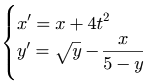The boundary conditions are: `x(0)=1, y(0)=4, t(0)=0, t(f)=1`.

This is the data entry to solve the problem:

d(x)/d(t) = x + 4*t^2 d(y)/d(t) = sqrt(y) - x/(5-y) x(0)=1 y(0)=4 t(0)=0 t(f)=1

or you may define any number of auxiliary expressions, to simplify long and/or repeating sections:

d(x)/d(t) = x + 4*t^2 d(y)/d(t) = sqrt(y) - a a = x/(5-y) x(0)=1 y(0)=4 t(0)=0 t(f)=1

And the resulting report would look like: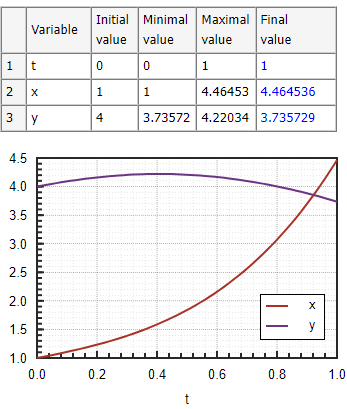The problems belonging to this category are comprised of systems of simultaneous first-order ordinary differential equations and explicit algebraic equations. The differential equations must be entered in the following form:
`d(x)/d(t) = an expression `

Here t is the independent variable and x is a dependent variable. Note that other independent and dependent variable names are possible. Auxiliary algebraic equations must be entered in the form:

`x = an expression`

where the same naming rules apply.

An expression may contain any combination of constants and variables. However, the auxiliary equations must be explicit (not simultaneous). PolymathPlus will sort and order the equations and will indicate if a simultaneous situation exists (by warning of 'circular dependency').

Initial conditions must be specified for all the variables defined by differential equations, as well as the independent variable. A final value must also be specified for the independent variable.

# Set of consecutive reactions d(A)/d(t) = -k1*A d(B)/d(t) = k1*A - k2*B d(C)/d(t) = k2*B k1 = 1 k2 = 2 A(0) = 1 B(0) = 0 C(0) = 0 t(0) = 0 t(f) = 3

The Solution Report includes a Table listing the initial, minimal, maximal, and final values of all the variables over the integration range. In the example shown, the final values are: A = 0.049787, B = 0.047308 and C = 0.902905. A table containing 50 values of the dependent variables between the initial and final values of the independent variable is also displayed.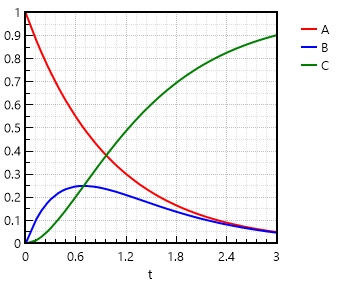Note that the same ODE problem can also be entered in a simplified format such as this:

# Set of consecutive reactions A' = -k1*A B' = k1*A - k2*B C' = k2*B k1 = 1 k2 = 2 A|1 B|0 C|0 t|0:3

## Regressions of Data  👆

This category of problems involves the fitting of data to various mathematical expressions.  The resulting fitted expressions can be considered by their ability to statistically represent the data.
PolymathPlus allows the user to fit data sets to

The known data points should be entered in a strict tabulated form enclosed in square brackets `[ ]`. Open bracket `[` and close bracket `]` should get their own dedicated lines.
The 1st line in the table contains the variable names separated by spaces. The rest of the lines contain the values of the variables, columns are seperated by spaces. The number of columns in each line must match, blank values are not allowed.
For example:

[ a b xy 1 9 1.1 2 13 7.87 3.1 16 5.67 4.2 18.2 8.9 5 19 45.6 6 20 56.7 7 19 66.9 ]

Tip: Use the Edit > Align Table menu item to align the columns nicely.

## Polynomial Regression 👆

PolymathPlus can fit a polynomial of degree n with the general form:

y = P(x) = a0 + a1x + a2x2 + . . . + anxn

or a linear equation when the equivalent first degree polynomial is specified

y = P(x) = a0 + a1x

where a0, a1, ..., an are regression parameters to a set of N tabulated values of x (a single independent variable) versus y (a single dependent variable). The highest degree allowed for a polynomial is N - 1 (thus n >= N - 1). The program calculates the coefficients a0, a1, ..., an by minimizing the sum of squares of the deviations between the calculated y or P(x) and the data for y.

A polynomial regression is specified by the "polyfit" command that must be followed by the name of the independent variable vector, name of the dependent variable vector, a number representing the degree of the polynomial, and an optional string 'origin' which indicates that the polynomial regression curve must go through the origin. These names must be separated by commas.

#### Correlation of BOD data example

Consider the following BOD (biological oxygen demand) data versus Time:

Time BOD days mg/liter ---- -------- 1 0.6 2 0.7 4 1.5 6 1.9 8 2.1 10 2.6 12 2.9 14 3.7 16 3.5 18 3.7 20 3.8

Fitting a straight line (linear regression) to the BOD vs. Time data. `BOD = a0 + a1*Time`

# Straight line fit [ Time BOD 1 0.6 2 0.7 4 1.5 6 1.9 8 2.1 10 2.6 12 2.9 14 3.7 16 3.5 18 3.7 20 3.8 ] polyfit Time BOD 1

This program yields the results a0 = 0.657797 (±0.374896), (95% confidence intervals shown between parentheses), a1 = 0.178056 (±0.031674), R2 = 0.9472735 and the variance: σ2 = 0.0825304.

In the Solution Report a table containing  the observed (measured) y values, the predicted y values (ycalc) and the residuals (r = y- ycalc) are also displayed.

Fitting the data to a 2nd degree polynomial.
To fit a 2nd degree polynomial, the polyfit statement is revised:

polyfit Time BOD 2

The results obtained in this case are a0 = 0.224387 (±0.353054 ), a1 = 0.310403 (±0.081692 ), a2 = -0.006439 (±0.003851), R2 = 0.9815572 and σ2 = 0.0324762.

To fit a 2nd degree polynomial which passes through the origin, the following polyfit statement is used:

polyfit Time BOD 2 origin

For this polynomial a0 = 0, by definition, and the rest of the results are: a1 = 0.354779 (±0.044171), a2 = -0.008236 (±0.002724), R2 = 0.9766053 and σ2 = 0.0366186.

Below are the plots containing: BOD (the data) vs. P(x) (calculated values), and Delta BOD (residuals) vs. time.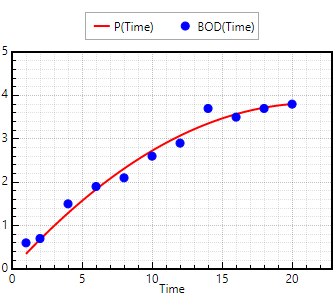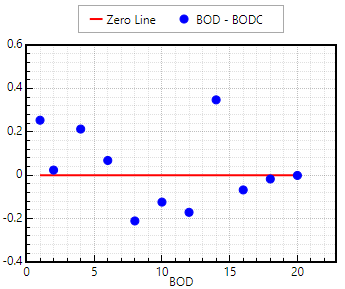Regression Analysis: Judging by the R2 and σ2 values, the 2nd degree polynomial represents the data the best. However, the confidence interval value of a0 shows that this parameter is not significantly different from zero, thus the use of 2nd degree polynomial passing through the origin should be preferred.

Consider the following set of Pressure (mm Hg) versus Temperature (deg C) data:

Temperature, T Pressure, P (Deg. C) (mm Hg) --------------- --------------- -36.7 1 -19.6 5 -11.5 10 -2.6 20 7.6 40 15.4 60 26.1 100 42.2 200 60.6 400 80.1 760

The following operations need to be carried out with these data:

1. Convert the temperature to K (by adding 273.15 to the value shown)
2. Convert the pressure to kPa (by multiplying the value shown by 0.1333)
3. Fit a fourth degree polynomial to the Pressure (dependent variable) vs. Temperature data.

The program to accomplish these tasks is shown below.

# Vapor Pressure Correlation [ T_C P_mmHg -36.7 1 -19.6 5 -11.5 10 -2.6 20 7.6 40 15.4 60 26.1 100 42.2 200 60.6 400 80.1 760 ] T_K = T_C+273.15 P_kPa = P_mmHg*0.1333 polyfit T_K P_kPa 4

The Solution Report includes a Table listing the parameter values and confidence intervals on these values.
For this particular problem the following results are shown.

Variable Value Conf Interval --------- ----------- ------------- a0 2121.85 434.312 a1 -33.1504 6.01561 a2 0.196174 0.0310526 a3 -0.000522 7.080E-05 a4 5.28E-07 6.017E-08

The regression plot: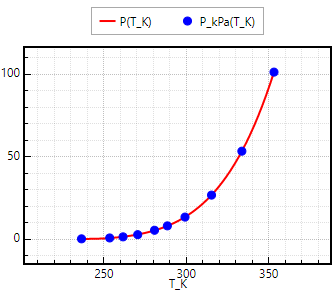The Solution Report includes also the statistical metrics: R2, R2adj, root mean squared deviation and variance. A table displaying the observed (measured) y values, the predicted y values (ycalc) and the residuals (r = y - ycalc).

To fit a polynomial that passes through the origin the "polyfit" command must also include the parameter "origin".
For example:

polyfit T_K P_kPa 4 origin

## Multiple linear Regression  👆

This PolymathPlus option will fit a linear function of the form:

y(x1, x2, ..., xn) = a0 + ax1 + ax2 + ... + axn

where a0, a1, ..., an are regression parameters, to a set of N tabulated values of x1, x2, ..., xn (independent variables) versus y (dependent variable). Note that the number of data points must be greater than n+1 (thus N >= n+1). The program calculates the coefficients a0, a1, ..., an by minimizing the sum of squares of the deviations between the calculated and the data for y.

Use the mlinfit command to request a multiple linear regression fit. The arguments are the independent variables vector's names, followed by the independent vector name. Additional optional (last) argument is the string 'origin' which indicates that the regression curve should pass through the origin (thus a0 = 0).

#### Simple multiple linear regression example

The following data are for the wear of a bearing y that may be dependent upon x1 = oil viscosity and x2 = load data.:

y x1 x2 --- ---- ---- 293 1.61 851 230 15.5 820 172 22 1058 91 45 1201 125 33 1357 125 40 1115

In this example we compare the linear regression models that includes x1 and x2 as independent variables, with a model that includes only x1.

`y = a0 + a1*x1 + a2*x2`

# Multiple linear regression [ y x1 x2 293 1.61 851 230 15.5 820 172 22 1058 91 45 1201 125 33 1357 125 40 1115 ] mlinfit x1 x2 y

The results obtained in this case are a0 = 360.836 (±118.076), a1 = -3.75246(±1.774), a2 = -0.084265(± 0.140313), R2 = 0.9835209 and σ2 = 159.1951.

To remove x2 from the regression model, the mlinfit statement is revised:

mlinfit x1 y

After this modification the following results are obtained: a0 = 292.784(±37.3385 ), a1 = -4.58727(±1.23994), R2 = 0.963462 and σ2 = 264.7302 .
Judging by the ``` ``` R2 ``` and ``` σ2 ``` ``` values, the regression model that contains both x1 and x2 represents the data better. However, the confidence interval value of a2 shows that this parameter is not significantly different from zero, thus removal of x2 from the model should be preferred `.`

Considering the "vapor pressure" example to fit a regression model of the form: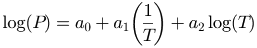The dependent variable can be defined by `logP=log(P_kPa)` and the independent variables defined by `OneonT=1/T_K` and `logT=log(T_K)`. Then `mlinfit` can be used to find the parameter values.

# VP Correlation by Reduced Riedel Eqs [ T_C P_mmHg -36.7 1 -19.6 5 -11.5 10 -2.6 20 7.6 40 15.4 60 26.1 100 42.2 200 60.6 400 80.1 760 ] # auxiliary variables derived T_K = T_C+273.15 P_kPa = P_mmHg*0.1333 logP = log(P_kPa) OneonT = 1/T_K logT = log(T_K) # regression model mlinfit OneonT logT logP

The Solution Report includes a Table listing the parameter values and confidence intervals on these values. For this particular problem the following results are shown.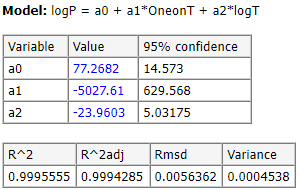## Nonlinear Regression  👆

Nonlinear regression involves a general mathematical function (model) of the form:

y = f (x1, x2, ..., xn, a0, a1, a2,...,am)

where a0, a1, ..., an are regression parameters to a set of N tabulated values of x1, x2, ..., xn (independent variables) versus y (dependent variable). Note that the number of data points must be greater than m + 1 (thus N >= m + 1).

Use the nlinfit command to request nonlinear regression fit. The argument is the nonlinear regression model: the dependent variable vector name on the left side of the "=" sign, the independent variable vectors names and the parameters on the right side. Initial estimates must be provided for all the parameter values using expressions of the form:

m(ai) = value

Fitting a nonlinear equation (Antoine) to the "vapor pressure example". The regression model is of the following form: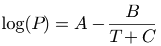where P is pressure (mmHg) , T is temperature (deg C) and A, B and C are parameters, need to be fitted to the "vapor pressure" example's data.
Following is the program for finding the optimal parameter values with nlinfit.

# Nonlinear Regression # Antoine Equation parameters [ T P -36.7 1 -19.6 5 -11.5 10 -2.6 20 7.6 40 15.4 60 26.1 100 42.2 200 60.6 400 80.1 760 ] logP = ln(P) # regression model nlinfit logP = A-B/(C+T) # initial guess for A, B, C m(A) = 8 m(B) = 2000 m(C) = 200

The optimal solution obtained is A = 5.76735, B = 677.094 and C = 153.885.

## Regression Statistics  👆

PolymathPlus provides statistical reports for the regression fit which assess the validity and accuracy of the regression. Each fitting variable has a 95% confidence interval. The confidence interval should be smaller than the absolute value of the variable for statistical validity.

In addition the following calculations are provided: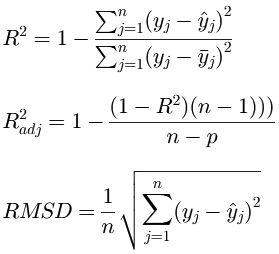The following formulas calculate variables, standard deviation, and chi squared.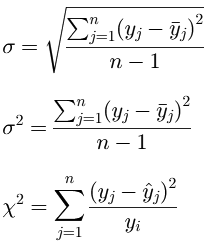The following formulas calculate MAE, MSE, RMSE.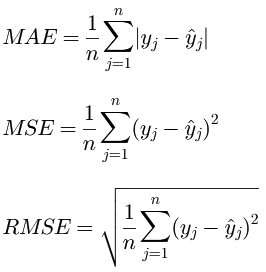# Variables and Expressions  👆

The following objects compose a valid PolymathPlus math expression:

 Expression Objects Description Number A number can be represented in decimal notation or engineering notation. Engineering notation can be used to indicate powers of 10 using 'e' or 'E' For example, 0.123e-5 is the same as 0.123*10^(-5). Note that only the period "." is used as the decimal delimiter symbol and comma nor spaces must not be used to indicate digits grouping. Sample valid PolymathPlus numbers: 1.0, 2300, 5E-7, 1.23E+9, 34.0046 Invalid PolymathPlus numbers: 5,000.00 12,9 12 000 000 Variable Name Variable names must begin with an alphabetical character and can contain alphabetic and numeric characters or underscore "_". Variable names are case-sensitive, i.e. 't' and 'T' are two different variables. Special characters such as \$, &, #, @ etc. are not allowed. Samples: x1, x2, A12, T, tm4eee, VH2O, PmmHg, KL_1 Arithmetic Operator Returns the arithmetic operations of the binary components. For example '2+3' returns 5 The '^' symbol is used for exponentiation. The +, -, *, / symbols are used for addition, subtraction, multiplication, and division respectively. The precedence of operators is ^, (* or /), (+ or -) which is invoked working from left to right in an expression. Samples: +, -, *, /, ^ (1 + 2 * 3 ^ 2 ^ 3 / 2 - 4) is equivalent to (1 + (2 * (((3 ^ 2) ^ 3) / 2)) - 4) Function Name Various mathematical function names are available. Examples: sin, cos, exp, ... The full list of available PolymathPlus functions is presented below. Samples: 12+sin(4) exp( 5*9+log(8)) Condition Operator These operators return "1" if the condition met, and "0" if the condition does not meet. Examples: The expression '3>6' returns 0. The expression '4>=4' returns 1. Valid conditional operators: >, <, >=, <=, == Boolean Operator The Boolean operators return "1" if the condition is met, and "0" if the condition is not met. Example: The expression '(2>3) or (1>0)' returns 1. The expression '1 and 0' returns 0. Valid Boolean operators: And, Or If-Then-Else There are three case-insensitive keywords which compose the if-then-else expression structure.  The three keywords are: If, Then, Else. Samples: If (x>5) Then (sin(12)+4) Else (sin(12)+8) if (x>5) then (sin(12)+4) else (sin(12)+8)

A special `if` statement is available, with the following syntax:
if (condition) then (expression1) else (expression2)

The parentheses are optional.
The condition may include the following operators: and, or (Boolean operators), > (greater than), < (less than), >= (greater than or equal), <= (less than or equal), == (equals).

The expressions may be any formula, including another `if` statement (nested statements).

For example:

A = if (x>0) then (log(x)) else(0) b = if (T<minT) then (minT) else (if (T>maxT) then (maxT) else (T)) Vol_h1 = if (a>5 and c<2) then 1.12 else 7.89

## Available Functions  👆

A number of standard functions are available for use in the various programs. The arguments of the functions must be enclosed in parentheses. The arguments may be themselves expressions or other functions. The nesting of functions is allowed.

All function names must be given in lower-case letters. The trigonometric functions require that their arguments be given in radians. Conversely, the inverse trigonometric functions give their results in radians.

You should note that the functions require that their arguments be enclosed in parentheses, but that the arguments do not have to be simple numbers. You may have a complicated expression as the argument for a function, and you may even nest the functions, using one function (or an expression including one or more functions) as the argument for another.

## Settings and Hints  👆

PolymathPlus allows for custom tuning of the solution algorithms, as well as basic adjustments to the output solution report. Settings are the default parameters and apply to all solution programs. Specific program parameters can be overridden by hints. Settings are generally stored in a dedicated settings file, while hints are program-specific and are stored as part of the program `*.pol`.

• Settings are default parameters which apply to all programs
• Hints are optional per program tuning which can override the default settings and are saved as part of the program itself
The default settings can be altered in the settings window. Hit the Save button for the changes to be saved.
To copy the set of available settings/hints selected the relevant advanced option from the Edit menu.

The main settings and hints apply to:

• Solution algorithms and error tolerance tuning
• Report display parameters such as displaying titles, charts, statistics
• Report display tuning such as chart size or significant digits.

## List of Global Settings  👆

# Setting Name Default Type
2 Report_fix_digits 10 Integer
3 Report_verbose No Boolean
4 Report_show_Source Yes Boolean
5 Report_show_Equations No Boolean
6 Report_show_charts Yes Boolean
7 Report_chart_height 350 Integer
8 Report_chart_width 450 Integer
9 Report_formatted_equations Yes Boolean
10 Report_formatted_equations_color No Boolean
11 Report_formatted_equations_scale 1.8 Number
12 NLE_SOLUTION_METHOD_INDEX 1 Integer
13 NLE_tol_x 1E-07 Number
14 NLE_tol_f 1E-07 Number
15 NLE_min_tol 1E-07 Number
16 NLE_eps 1E-07 Number
17 NLE_max_iter 150 Integer
18 DEQ_SOLUTION_METHOD_INDEX 0 Integer
19 DEQ_Intermediate_data_points 50 Integer
20 DEQ_RKF_H 1E-06 Number
21 DEQ_RKF_TETOL 1E-06 Number
22 DEQ_EPS 1E-05 Number
23 DEQ_H1 0.001 Number
24 DEQ_HMIN 1E-08 Number
25 NLR_SOLUTION_METHOD_INDEX 1 Integer
26 NLR_maxiter 64 Integer
27 NLR_tol 0.0001 Number
28 APP_WINDOW_WIDTH 1200 Integer
29 APP_WINDOW_HEIGHT 800 Integer
30 APP_WINDOW_MAXIMIZED No Boolean

Hints are pre-fixed with `#@` in the program file.
The following sample DEQ problem has a hint line to propose the size of the chart on the solution report:

#@ Chart_Size = 350; 300 d(x)/d(t) = x + 4*t^2 d(y)/d(t) = sqrt(y) - x/(5-y) t(0)=0 t(f)=1 x(0)=1 y(0)=4

## Linear Equations Programs Hints  👆

# Hint syntax sample & Description
1 `#@Report_fix_digits = 8`
Significant digits to display when presenting numbers
2 `#@Report_show_header = no`
Indicates whether a header text is displayed in the solution report
Valid values are yes or no
3 `#@Report_show_Settings = no`
Valid values are yes or no
4 `#@Report_show_Matlab = no`
Indicates whether a Matlab formatted problem should be displayed in the solution report
Valid values are yes or no

## Nonlinear Equations Programs Hints  👆

# Hint syntax sample & Description
1 `#@Report_fix_digits = 8`
Significant digits to display when presenting numbers
2 `#@Report_show_header = no`
Indicates whether a header text is displayed in the solution report
3 `#@Report_show_charts = no`
Indicates whether charts should be displayed in the solution report
Valid values are yes or no
4 `#@Report_show_Matlab = no`
Indicates whether a Matlab formatted problem should be displayed in the solution report
Valid values are yes or no
5 `#@Report_show_root_function_values = no`
Valid values are yes or no
6 `#@Report_show_Settings = no`
Valid values are yes or no
7 `#@Chart_Size = 400; 450`
Indicates the size of the chart to be drawn in the report
Values are Width; Height
8 `#@NLE_SOLUTION_METHOD_INDEX = 1`
Algorithm used to solve the NLE system. Valid method indexes are:
0=Fast-Newton, 1=Safe-Newton, 2=Safe-Broydn, 3=Constrained
9 `#@NLE_tol_x = 1E-07`
10 `#@NLE_tol_f = 1E-07`
11 `#@NLE_min_tol = 1E-07`
12 `#@NLE_eps = 1E-07`
13 `#@NLE_max_iter = 150`

## Differential Equations Programs Hints  👆

# Hint syntax sample & Description
1 `#@Report_fix_digits = 8`
Significant digits to display when presenting numbers
2 `#@Report_show_header = no`
Indicates whether a header text is displayed in the solution report
3 `#@Report_show_charts = no`
Indicates whether charts should be displayed in the solution report
Valid values are yes or no
4 `#@Report_show_Matlab = no`
Indicates whether a Matlab formatted problem should be displayed in the solution report
Valid values are yes or no
5 `#@Report_show_Settings = no`
Valid values are yes or no
6 `#@Report_Show_Intermediate_data_points = no`
Valid values are yes or no
7 `#@DEQ_Intermediate_data_points = 50`
8 `#@DEQ_RKF_H = 1E-06`
9 `#@DEQ_RKF_TETOL = 1E-06`
10 `#@DEQ_EPS = 1E-05`
11 `#@DEQ_H1 = 0.001`
12 `#@DEQ_HMIN = 1E-08`
13 `#@DEQ_SOLUTION_METHOD_INDEX = 3`
DEQ solution method algorithm. Valid indexes are:
0=RKF45, 1=RKF56, 2=BS, 3=Stiff, 4=StiffBS
14 `#@Chart_Size = 400; 450`
Indicates the size of the chart to be drawn in the report
Values are Width; Height
15 `#@Chart_Y_Curves = A; B; k3; k4; k5`
Indicates the variables names, separated by semicolon, to be drawn on the solution graph.
Invalid names will be ignored. You should replace the default entries.
16 `#@Chart_GridLines = 1; 1; 1; 1`
Indicates the gridlines width. Width 0 is essentially no gridlines. Values are:
MajorX; MinorX; MajorY; MinorY
17 `#@Chart_X_Scale = 0; 11; 1; 0.2`
Indicates the X axis scale. Values are (steps and padding values are optional):
Minimum value; Maximum value; Major step; Minor step; Minimum padding; Maximum padding
18 `#@Chart_Y_Scale = -30; 380; 50; 10; 0.3; 0.3`
Indicates the X axis scale. Values are (steps and padding values are optional):
Minimum value; Maximum value; Major step; Minor step; Minimum padding; Maximum padding
19 `#@Chart_Title = `
20 `#@Chart_SubTitle = `
21 `#@Chart_X_Axis_Title = x (min)`
22 `#@Chart_Grayscale = no`
Valid values are yes or no
23 `#@Chart_inner_background_html_color = #FFFFFF`
24 `#@Chart_outer_background_html_color = #FFFFFF`
25 `#@Chart_text_font_size = 12`

## Regression Programs Hints  👆

# Hint syntax sample & Description
1 `#@Report_fix_digits = 8`
Significant digits to display when presenting numbers
2 `#@Report_show_header = no`
Indicates whether a header text is displayed in the solution report
3 `#@Report_show_data_points = no`
Display calculated datapoints table
Valid values are yes or no
4 `#@Report_show_settings = no`
Show program settings
Valid values are yes or no
5 `#@Report_show_charts = no`
Indicates whether charts should be displayed in the solution report
Valid values are yes or no
6 `#@Report_show_Matlab = no`
Indicates whether a Matlab formatted problem should be displayed in the solution report
Valid values are yes or no
7 `#@Chart_Size = 400; 450`
Indicates the size of the chart to be drawn in the report
Values are Width; Height
8 `#@Chart_GridLines = 1; 1; 1; 1`
Indicates the gridlines width. Width 0 is essentially no gridlines. Values are:
MajorX; MinorX; MajorY; MinorY
9 `#@Chart_X_Scale = 0; 11; 1; 0.2`
Indicates the X axis scale. Values are (steps and padding values are optional):
Minimum value; Maximum value; Major step; Minor step; Minimum padding; Maximum padding
10 `#@Chart_Y_Scale = -30; 380; 50; 10; 0.3; 0.3`
Indicates the X axis scale. Values are (steps and padding values are optional):
Minimum value; Maximum value; Major step; Minor step; Minimum padding; Maximum padding
11 `#@Chart_Title = `
12 `#@Chart_SubTitle = `
13 `#@Chart_X_Axis_Title = x`
14 `#@Chart_inner_background_html_color = #FFFFFF`
15 `#@Chart_outer_background_html_color = #F5F5F5`
16 `#@Chart_text_font_size = 12`
17 `#@Chart2_Size = 200; 150`
18 `#@Chart2_X_GridLines = yes`
Valid values are yes or no
19 `#@Chart2_Y_GridLines = yes`
Valid values are yes or no
20 `#@Chart2_X_Scale = 0; 11; 1`
21 `#@Chart2_Y_Scale = -1; 1; 0.2`
22 `#@Chart2_Title = `
23 `#@Chart2_SubTitle = `
24 `#@Chart2_X_Axis_Title = x`
25 `#@Chart2_inner_background_html_color = #FFFFFF`
26 `#@Chart2_outer_background_html_color = #F5F5F5`
27 `#@Chart2_text_font_size = 12`
28 `#@NLR_SOLUTION_METHOD_INDEX = 1`
Algorithm used to solve the NLR model. Valid method indexes are:
0=MRQMIN, 1=L-M
29 `#@NLR_maxiter = 64`
30 `#@NLR_tol = 0.0001`